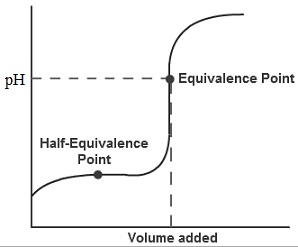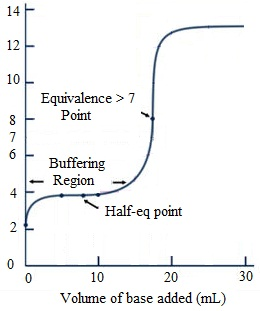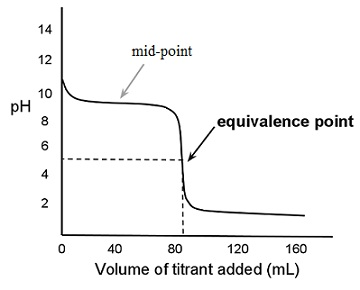#### Ionic equilibria in weak acids and bases, Chemistry tutorial

Introduction:

All the weak acids in solution exist in equilibrium. The equilibrium is in between the molecular form of acid and the ionized form of the acid.

HA → H+ (aq) + A- (aq)

For all the weak acids this equilibrium lies mostly on the left. Most of the acid is in the molecular form. This yields in all weak acids encompassing an equilibrium constant which is less than 1. The highest equilibrium constant is around 10-2. The smallest is around 10-13. The equilibrium expression for all the monoprotic weak acids is:

Ka = [H+][A-]/[HA]

Here, Ka is the acid-ionization constant.

Determination of Ka:

Ka can be found out in a variety of manners. The most general is by measuring the pH of a solution of the weak acid in question. This process works fine for the monoprotic acids, however for polyprotic acids; the outcome is a combination of the different K's for each and every acidic proton. Titration is a procedure which works well for either monoprotic or polyprotic acids. Measuring the pH at different points in the titration and plotting the pH versus the volume of the base added provides an indication of the K's for the acid.

Illustrations:

A 0.10 M weak acid consists of a pH of 4.56. Determine the Ka for the acid?

[H+] = 10-pH = 10-4.56 = 2.8 x 10-5 M

 HA H+ A- Initial [] 0.10 10-7 0 Δ -x +x +x Final [] 0.10 - x 10-7 + x x

(10-7 + x) is the equilibrium hydrogen ion concentration that is 2.8 x 10-5 M.

This is as well equivalent to the [A-] due to the Stoichiometry of the problem. The [HA] at equilibrium is necessarily similar as it was at the starting. This provides a Ka of:

Ka = [H+][A-]/[HA] = (2.8 x 10-5)2/0.10 = 7.6 x 10-9

The acid is a fairly weak acid.

Using Ka:

The use of Ka is just similar to the use of any other equilibrium constant. We can make use of it to determine the equilibrium concentrations of everything in the solution or just one thing, generally Hydrogen ion. The processes of solving a weak acid (or weak base) equilibrium are the similar as for any other kind of equilibrium. Generally we are capable to make some approximations to the problem which makes the mathematics easier to resolve. At times, we just have to work our way via (generally) quadratic equation that comes up. The thing to remember regarding this is that these are still equilibrium problems. They are no different than any other equilibrium problem we have done prior to.

Illustrations:

Compute the pH of a 0.15 M solution of Acetic acid. Ka = 1.8 x 10-5.

Set up the problem the similar manner.

This provides an equilibrium expression which looks like:

Ka = [10-7 + x] [x]/[0.15 - x] = 1.8 x 10-5

The quantity we are looking for is 10-7 + x. We can make several guesses at approximation although. For illustration, x is probably quite small as this is a weak acid. Thus, we can probably ignore it in comparison to 0.15. As well, it is an acid so that the amount of Hydrogen ion generated is probable to be much bigger than the amount from the auto-dissociation of water thus we can ignore the 10-7 in relation to x. This provides a simplified equilibrium expression that looks similar to:

Ka = [x][x]/0.15 = 1.8 x 10-5

This can be resolved relatively (as compared to the full quadratic) simply.

Ka ≈ [x][x]/0.15 = 1.8 x 10-5

x2/0.15 ≈ 1.8 x 10-5

x2 ≈ 1.8 x 10-5 x 0.15 = 2.7 x 10-6

x ≈ √ (2.7 x 10-6) = 1.6 x 10-3 ≈ [H+]

Now we require checking our suppositions. Is this value much less than 0.15? If we subtract this from 0.15 we get 0.1484, which in the significant figures of the problem is necessarily 0.15. What about the supposition that it is much larger than 10-7 ? This value plus 10-7 is 1.6001 x 10-3. Again, in the significant figures of the problem, this is 1.6 x 10-3. We can now make use of this value to compute the pH that is:

pH = -log [H+] = - log (1.6 x 10-3) = 2.80

Polyprotic acids:

Most of the polyprotic acids are weak. The exemption is the first proton to come off sulphuric acid, which is strong. A polyprotic acid consists of a Ka for each and every acidic proton on the acid. Each and every successive Ka is smaller than the prior one. Given that there is more than one place for the protons to enter the solution, how do we handle the computation of the pH of such a solution? The answer is simpler than one would think. We can handle each of the proton ionizations as the separate problems. The outcomes from the prior one are the initial concentrations for the later.

Illustration:

Determine the pH of a 0.10 M Phosphoric acid solution?

The three equilibria are:

H3PO4    → H+ + H2PO4-    Ka1 = 6.9 x 10-3

H2PO4-   → H+ + HPO42-    Ka2 = 6.2 x 10-8

HPO42-   → H+ + PO43-       Ka3 = 4.8 x 10-13

We resolve the first equilibrium. If we make use of the quadratic equation to solve this problem, we obtain [H+] = [H2PO4-] = 0.023. This then becomes the initial conditions for the second ionization.

 H2PO4- H+ HPO42- Initial [] 0.023 0.023 0 Δ -x +x +x Final [] 0.023 - x 0.023 + x x

Whenever we make some simplifying suppositions here, which are valid, we obtain the Hydrogen ion concentration similar as it is from the first ionization. The concentration of HPO42- ion is equivalent to Ka2. These then become the preliminary conditions for the third ionization.

 HPO4-2 H+ PO43- Initial [] Ka2 0.023 0 Δ -x +x +x Final [] Ka2- x 0.023 + x x

Solving this problem by employing the simplifying approximations provides that the Hydrogen ion and mono-hydrogen phosphate ion concentrations don't change considerably. The concentration of the phosphate ion is:

[PO43-] = Ka3 Ka2/[H+]

These outcomes will be similar for any triprotic weak acid.

Weak Bases:

Weak bases are nearly for all time derivatives of the Ammonia molecule (NH3). Weak bases in water experience hydrolysis, the breaking apart of water.

NH3 (aq) + H2O (l) → NH4+ (aq) + OH- (aq)

The equilibrium expression for this is:

Kb = [NH4+] [OH-]/[NH3]

Kb is termed as the base-ionization constant. The processes for finding out Kb are similar as those used to find out Ka. Kb can as well be employed to find out the pH of a solution of a weak base. There are no polyprotic weak bases which we are going to look at.

Illustration:

Compute the pH of a 0.25 M pyridine solution. Kb = 1.4 x 10-9.

Set up the problem the mode we would for any equilibrium.

 Py+ H2O PyH+- OH- Initial [] 0.25 0 10-7 Δ -x +x +x Final [] 0.25 - x x 0.25 + x

Kb = [PyH+][OH-]/[Py] = (x) (10-7 + x)/(0.25 - x)

We can try our suppositions here too.

Kb = [PyH+][OH-]/[Py] = (x) (10-7 + x)/(0.25 - x) ≈ x2/0.25 ≈ 1.4 x 10-9

x ≈ √[(0.25) (1.4 x 10-9)] = 1.9 x 10-5 ≈ [OH-]

Are the estimations valid? This value is just about the same as itself plus 10-7. As well it is much less than the value of 0.25, thus the approximations are valid. The pH can now be computed. First determine the Hydrogen ion concentration.

[H+] = Kw/[OH-] = (1.0 x 10-14)/(1.9 x 10-5) = 5.3 x 10-10

pH = - log (5.3 x 10-10) = 9.27

pH of a salt solution:

To determine the pH of a salt solution, we should first look at the ions which make up the salt.

a) When both ions come from a strong acid and base then the pH of the solution of that salt will be neutral (7.0).

b) When one of the ions comes from a weak acid, the pH will be basic (> 7.0) as the conjugate of the weak acid will be a weak base that will hydrolyze and produce Hydroxide ions.

c) When one of the ions comes from a weak base, the pH will be acidic (< 7.0) as the conjugate of a weak base is the weak acid. The weak acid will dissociate, generating Hydrogen ions.

d) If the ions come from both the weak acid and a weak base, the pH can be found out by determining which of the conjugates is stronger. If the conjugate acid is stronger than solution will be acidic. If the conjugate base is stronger, then the solution will be basic.

Buffers:

A solution which includes significant amounts of both a weak acid and its conjugate base (or vice-versa) before equilibrium is reached is a buffer. A buffer is a solution which will resist a change in pH on the addition of acid or base. This works as, in the solution, there is a significant amount of acid and base present to react by any base or acid that is added. This maintains the equilibrium at approximately similar point, keeping the pH relatively constant.

If we are capable to make some simplifying suppositions in the equilibrium computation, we can find out a simple relationship between the pH of a buffer solution and the ratio of the acid to its conjugate base. The relationship is based on the equilibrium constant for the acid in question.

pH = pKa + log ([base]/[acid]

This equation is termed as the Henderson-Hasselbalch equation. pKa is the negative of the common logarithm of the Ka. This relationship will hold if there is a buffer present. This is a simplification of the equilibrium computation. By using this equation or the full quadratic in the equilibrium computation must provide the similar results.

Titration Curves:

A) Strong acid-Strong base:

This curve is relatively simple. The pH begins at the pH of the strong solution. The pH of the end-point is for all time 7.0. The computations comprised are simple Stoichiometry computations.Fig: Strong acid-strong base

B) Weak acid-Strong base:

This curve is a little more fascinating. The pH begins at the pH of the weak acid. The pH at the end-point will for all time be above 7.0. The computations comprised here are the Stoichiometry and the equilibrium computations. Most of the way up to the end-point a buffer is present in such a way that the computations there will comprise the Henderson-Hasselbalch equation. At the point where the volume of base added is halfway to the end-point, the pH of the solution is equivalent to the pKa of the weak acid.Fig: Weak acid-Strong base

C) Weak base-Strong acid:

This curve is identical to the curve above. The pH begins at the pH of the weak base. The pH at the end-point here will for all time be below 7.0. Most of the way up to the end-point a buffer is present in such a way that the computations there will comprise the Henderson-Hasselbalch equation. At the point where the volume of base added is halfway to the end-point, the pH of the solution is equivalent to the pKa of the conjugate acid of the weak base.Fig: Weak base-Strong acid

Tutorsglobe: A way to secure high grade in your curriculum (Online Tutoring)